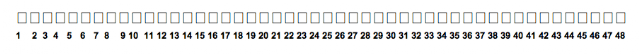## Sunday, July 29, 2012

### Fact Smart: Building Arrays with Google Table

Creating, examining and comparing arrays is a great way for fourth graders to become fact smart.

We'll build arrays using Google table.  Learning to use Google table at the start of the year gives students an awesome tool for problem solving and information organization.

We'll start by building all the arrays for 24 together--each child will have a computer, and I'll have my computer hooked up to the white board.
1. Students will open Google docs and create a document.
2. Students will name the document like this "John's Number Arrays."
3. We'll talk a bit about what an array is and then I'll ask students to name arrays for 24.
4. We'll build each array and color it in (use "table properties'). Later we'll discuss how arrays are named by their dimensions and their dimensions are the factor pairs for that number.
5. After that I'll have students work with partners to build all the possible arrays for 36, then 48.  As the numbers get larger, students may have to build the 1 X __ by inserting special characters from the menu bar, rather than the table tool as that has some size limitations (you can make a 1X20, then add several cells to it if you have the font reduced as much as possible).
There are many wonderful tools on Google and other programs that help students to organize and develop their mathematical thinking.  Introducing these tools to students early on provides them with a great resource with which to communicate and deepen mathematical understanding.I used the menu bar to insert 48 "special characters" for this lengthy array. As students complete arrays for 24 and 36, you can then hand out specific numbers for which children are responsible for creating all the arrays.  After that, the class can begin making a bulletin board filled with arrays for numbers from 1 to 100.  During a class discussion, you can then begin to discuss the following questions: 1. Which numbers have the most arrays (factor pairs)? 2. Which numbers have the least? 3. What do you notice about the numbers that have the least (all odd, except 2--prime numbers). 4. What do you notice about the numbers that have the most arrays? 5. Do we see any perfect numbers? 6. How many composite numbers do we have represented? 7. Do you notice any numbers that make a square?  Which numbers, and how do you know the array is a square? This careful attention to numbers will help students to look at numbers with greater understanding and the ability to predict with regard to the number's function in an equation of mathematical problem.